Definition

Gradient descent using Newton's method is a variant of gradient descent where the step size along the gradient descent is determined using Newton's method. In other words, we move the same way that we would move if we were applying Newton's method to the function restricted to the line of the gradient vector through the point.

By default, we are referring to gradient descent using one iteration of Newton's method, i.e., we stop Newton's method after one iteration. However, we can consider gradient descent using Newton's method where we use multiple iterations of Newton's method to determine the step size for gradient descent.

Learning algorithm

Explicitly, the learning algorithm is: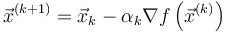$\vec{x}^{(k+1)} = \vec{x}_k - \alpha_k \nabla f\left(\vec{x}^{(k)}\right)$

where$\nabla f(\vec{x}_k)$ is the gradient vector of$f$ at the point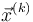$\vec{x}^{(k)}$ and$\alpha_k$ is the second derivative of$f$ along the gradient vector. Explicitly, if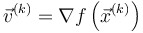$\vec{v}^{(k)} = \nabla f\left(\vec{x}^{(k)}\right)$, we have: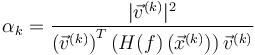$\alpha_k = \frac{|\vec{v}^{(k)}|^2}{\left(\vec{v}^{(k)}\right)^T\left(H(f)\left(\vec{x}^{(k)}\right)\right)\vec{v}^{(k)}}$

Here,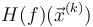$H(f)(\vec{x}^{(k)})$ is the Hessian matrix of$f$ at the point$\vec{x}^{(k)}$.

The expression in the denominator can be justified based on the fact that Hessian matrix defines bilinear form that outputs second-order directional derivatives.